## 5.2.1 Carbon Fluorides for Silicon Dioxide Etching

When modeling the plasma etching of silicon dioxide, it is important to include microloading phenomena which cause tapered vertical walls on the etched SiO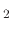profile . The tapering is caused by a polymer depositing on the well walls during the etching process. For example, when SiOis etched using CF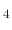gas, the CFgas undergoes a gas phase reaction, where it breaks into CFgas and F atoms. The CFgas then undergoes a surface deposition reaction with the SiO. In order to simulate this phenomenon in the presented LS framework, a model from  is implemented. It deals with an etching process with a simple chemistry such as a pure CFetch of SiOunder Ar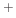bombardment and polymer inhibition. The model parameters and constants which are implemented here can be found in .

The model suggests three surface coverages, one for the etchant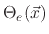, one for the polymer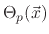, and one for the active sites on the polymer coverage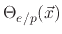. The coverages are found using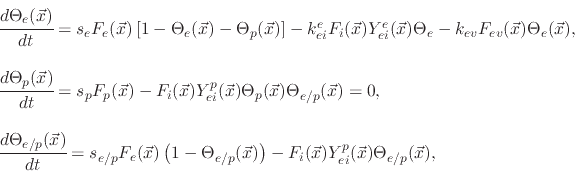(194)

where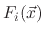,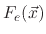, and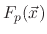are the total fluxes of the ion, etchant, and polymer, respectively and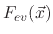is an evaporation flux which is proportional to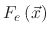and temperature dependent. The constants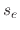,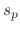, and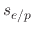are the sticking coefficients of the etchant on SiO, polymer on SiO, and etchant on the deposited polymer, respectively.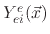and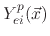are etching yield functions.

Assuming steady state conditions, (5.35) can be used to find the surface coverages for each particle species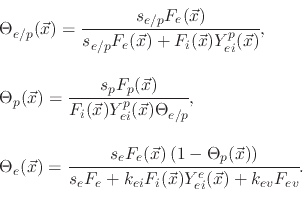(195)

The deposition rate of the polymer gas onto the wafer surface is given by the polymer flux and the sticking coefficient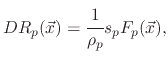(196)

whereis the polymer bulk density. The polymer can also be etched and this rate is given by the chemical sputtering rate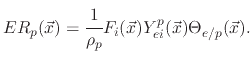(197)

If the polymer deposition rate is higher than its etch rate, then no etching on the surface occurs, but rather a deposition whose rate is given by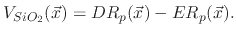(198)

However, if the polymer deposition rate is lower than its etch rate, the film etching rate is given by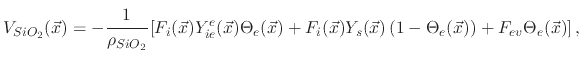(199)

where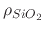is the SiObulk density and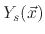accounts for the sputter yield of the silicon dioxide which is not covered by the etchant.

The threshold yield functions depend on the ion energies and the impact direction of the ions onto the surface. In the case of physical sputtering, the yield is given by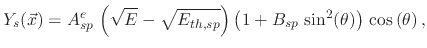(200)

whereis the impact angle,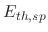is the sputtering threshold energy,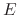is the ion impact energy, and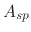is a sputtering yield factor. For ion enhanced chemical etching, the yield function is given by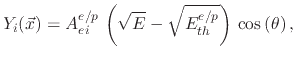(201)

where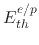is the threshold energy and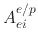the etchant yield factor for ion enhanced chemical etching.

Figure 5.6 shows a simulation of the silicon dioxide etching process when various ion, etchant, and polymer fluxes are used. It is clear that the ratio of etchant to polymer flux will decide how much polymer will be present on the sidewalls. Therefore, as the amount of polymer is decreased with respect to the amount of etchant, shown from Figure 5.6a to 5.6c, the sidewalls show an increasing vertical profile and higher etch rates. The simulation is performed through a 300nm diameter hole with an ideal mask for 80s. The profile is shown at 2s intervals. This means that when etching the silicon dioxide layers for BiCS memory holes, an etchant should be used which produces less deposition-inducing byproducts. When using CF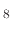, which was the etching process described in , the ratio of etchant to polymer inhibitor flux is shown to be 2.5:1.

Figure 5.6: Images showing the etched SiOtopography when using a fluorocarbon gas as the etchant, implemented using the described model.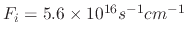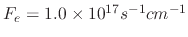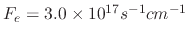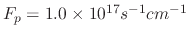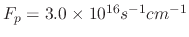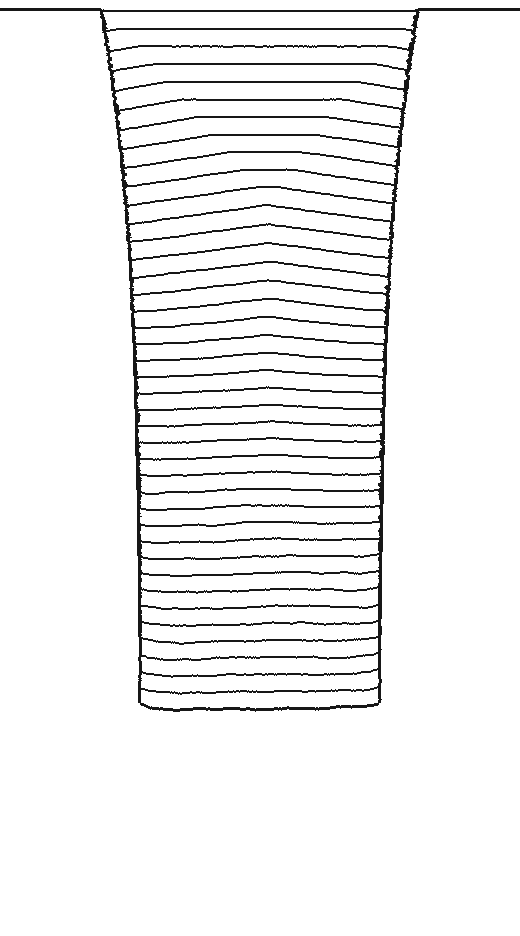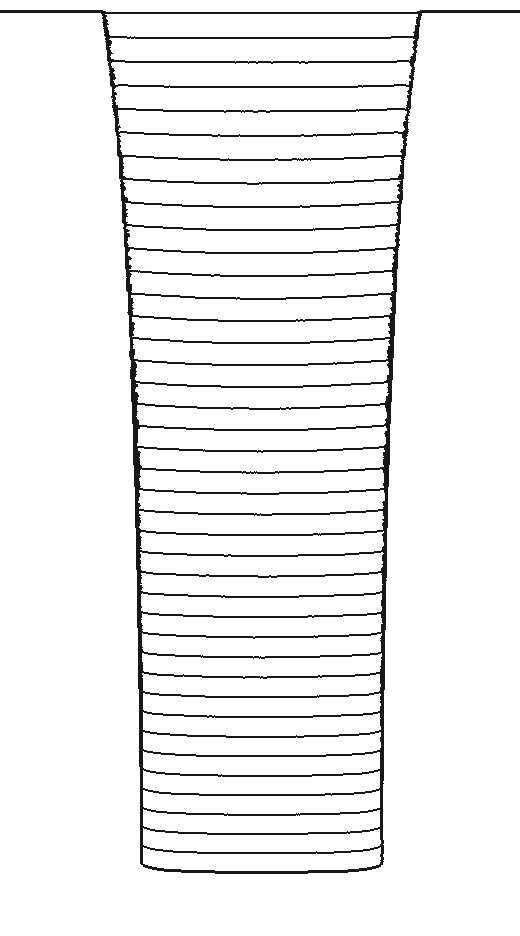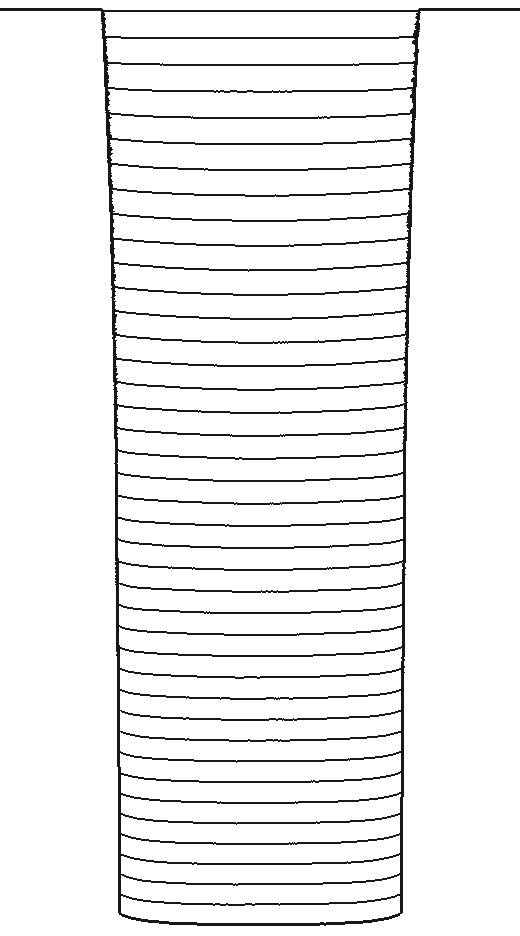The ratio of etchant to polymer flux is not the only process parameter governing the final etched profile. Another important factor is the sticking coefficient of the inhibitor species. The process described by the model in  suggests a sticking probability of 0.26. However, it would be beneficial for a process with a lower sticking probability to be used when sharp vertical etch profiles are required. In  a process using CFis suggested for SiOetching for BiCS memory holes, which has the deposition precursor CFwith a 0.0292 sticking probability. Figure 5.7 shows how varying the sticking probability influences the etched profile when the flux of ion, etchant, and polymer species are,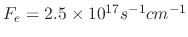, and, respectively. The simulations were performed through a 50nm opening, which is the approximate width required for etching BiCS memory holes, for 5s which should be sufficient for the required depth (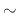33nm).

Figure 5.7: Images showing the etched SiOtopography when using fluorocarbon gas as the etchant for various polymer sticking coefficients.Coefficient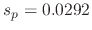Coefficient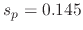CoefficientL. Filipovic: Topography Simulation of Novel Processing Techniques